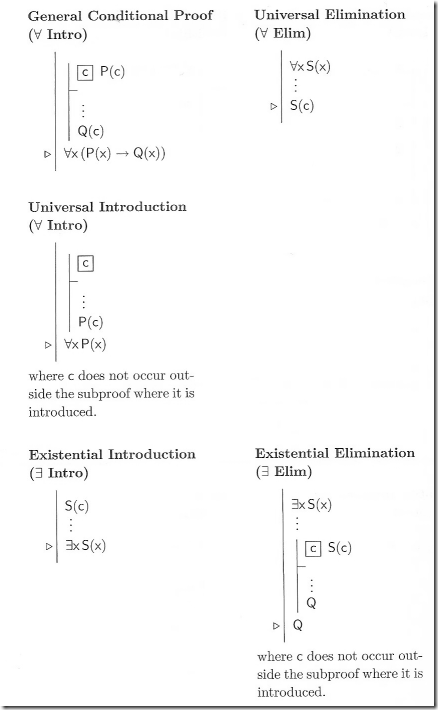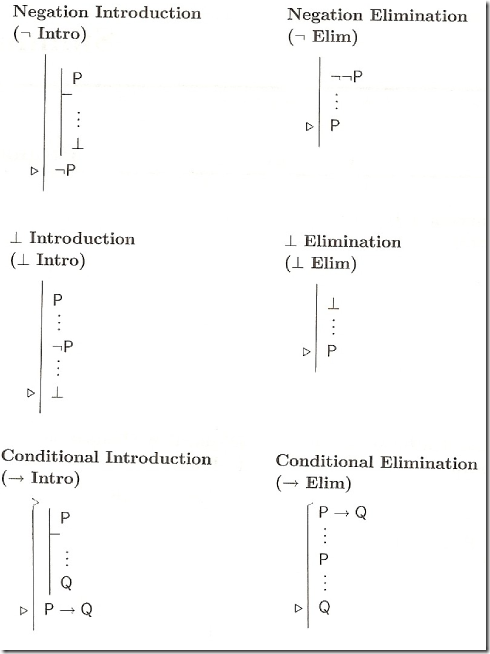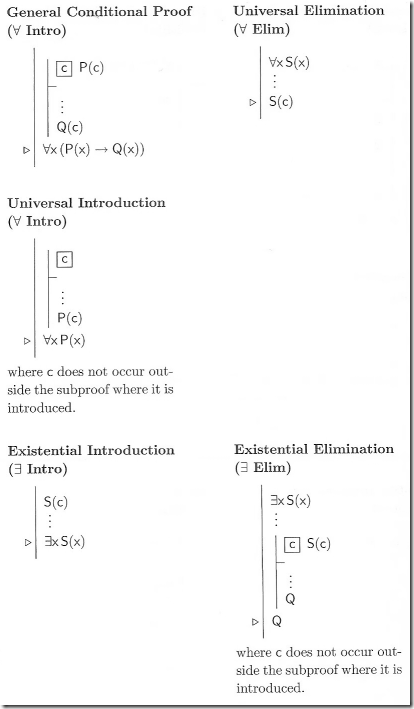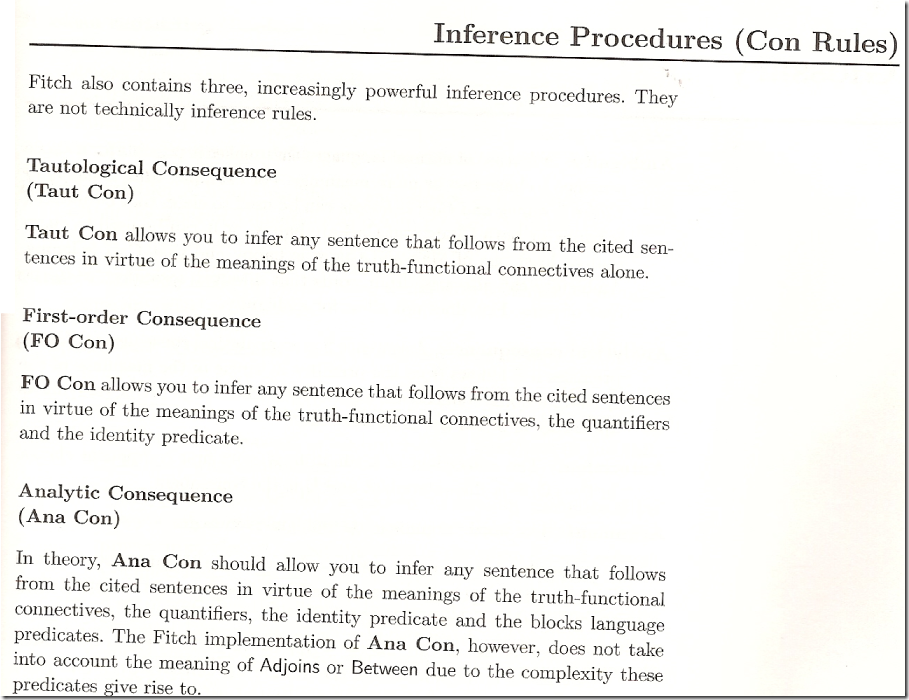Geeks With Blogs

Any ramblings and blog posts associated with the UNISA COS 261C tag should be considered study notes for my lectures...

Today I managed to start my revision for my Unisa Formal Logic 2nd Year Subject. It has been more than a semester since I did the original work and I have less than 30 days before the actual exam.

Below is an outline of the subject material as well as any exam related material for the subject.

### Outline of Subject

There will be 2 sections to the subject…

1. Propositional Logic
2. Quantifiers

Propositional Logic

• Atomic Sentences
• The logic of Atomic Sentences
• The Boolean Connectives
• The Logic of Boolean Connectives
• Methods of Proof for Boolean Logic
• Formal Proofs and Boolean Logic
• Conditionals
• The Logic of Conditionals

Quantifiers

• Introduction to Quantification
• The Logics of Quantifiers
• Multiple Quantifiers
• Methods of Proof for Quantifiers
• Formal Proofs and Quantifiers

### Mathematical Background

The subject has a require mathematical background – you will need to know at least the following mathematical sections…

Numbers

• Natural Numbers
• Integers
• Rational Numbers
• Real Numbers

Sets

• Sets, their elements and equality
• Notation for sets
• Subsets
• Operations on sets (Intersection, Union, Distributive Rules, Complement)
• Cartesian product, relations and functions (Ordered Pairs, Cartesian Product, Relations, Functions)

Recursion and Induction

• Definitions by recursion
• Proofs by induction

### Exam Outline

The 2010 Exam will be divided into two sections.

• Section A will be multiple choice and count for 24 marks
• Section B will be written and count for 76 marks

Examples of Questions from Section A that will have multiple choice answers include…

• Given a specific Tarski world and a number of sentences in the block language, you have to establish which of these sentences are not true in the given Tarski world and write down the option indicating that. The world will be specified in a way similar to the worlds given in assignment 1 of 2010
• Asked to write down the option giving correct English translation of an FOL sentence in the block language or a correct FOL translation in the block language of an English sentence.
• Required to derive a Disjunctive Normal Form or Conjunctive Normal Form of a given FOL sentence (as rough work) and then write down the option given a correct DNF or CNF.
• Asked to construct a truth table of an FOL sentence or a single joint truth table of a few FOL sentences and then right down the option giving the correct interpretation of the truth table.
• Required to inspect an argument and then write down the option giving the correct evaluation of the argument with respect to soundness and validity.

Section B will have 6 questions, most of which will have subsections. Examples of questions include…

• Translating English sentences to first-order logic (FOL) formulas or FOL sentences to English sentences using the predicates and names given in a table in the examination paper.
• Establishing whether given arguments are valid or sound and explaining the answers
• Giving informal proofs of a valid argument using proof by cases and/or proof by contradiction.
• Constructing a counterexample to a given argument.

Giving formal proofs using the rules of natural deduction. For these questions the following is important…

• The Fitch System uses these rules
• De Morgan’s laws are not part of natural deduction rules
• You may not use Taut Con or FO Con or Ana Con
• You will find a summary of the rules that you may use on pages 557 to 560 of the textbook.

### Pages 557 to 560 of Textbook### Past Exam Papers

Posted on Saturday, October 2, 2010 1:32 PM UNISA COS 261 Formal Logic | Back to top

Related Posts on Geeks With Blogs Matching Categories

Comments on this post: COS261C Formal Logic 2 – Introduction and Exam Outline